Precalculus : Solve an Isosceles Triangle By Dividing Into 2 Right Triangles

Example Questions

Example Question #1 : Solve An Isosceles Triangle By Dividing Into 2 Right Triangles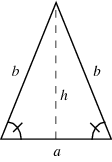Given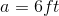, and the lower angles of the isosceles triangle are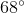, what is the length of? Round to the nearest tenth.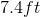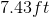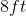Explanation:

Since the angle of the isosceles is, the larger angle of the right triangle formed byis also.

Using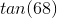, we can find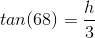.

Then solve for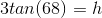.

Simplify: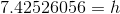.

Lastly, round and add appropriate units: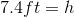.

Example Question #2 : Solve An Isosceles Triangle By Dividing Into 2 Right Triangles

In isosceles triangle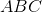,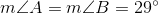.  If side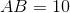, what is the approximate length of the two legs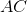and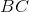?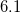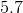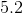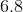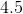Explanation: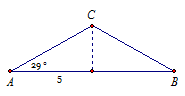In the diagram, AB is cut in half by the altitude.

From here it easy to use right triangle trigonometry to solve for AC.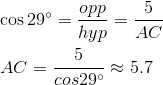Example Question #1 : Solve An Isosceles Triangle By Dividing Into 2 Right Triangles

Find the area of the following Isosceles triangle (units are in cm):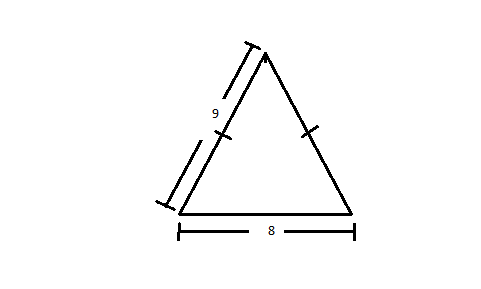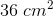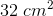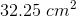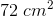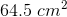Explanation:

The formula for the area of a triangle is: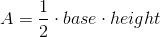We already know what the base is and we can find the height by dividing the isosceles triangle into 2 right triangles: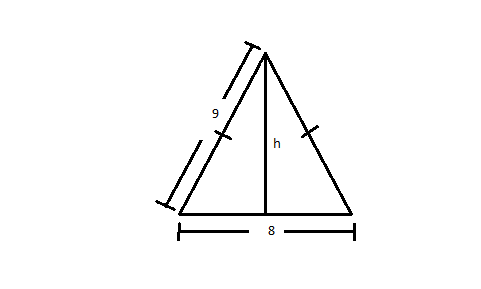From there, we can use the Pathegorean Theorem to calculate height: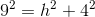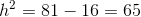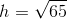To find the area, now we just plug these values into the formula: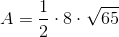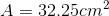Example Question #2 : Trigonometric Applications

Find the area of the given isosceles triangle and round all values to the nearest tenth: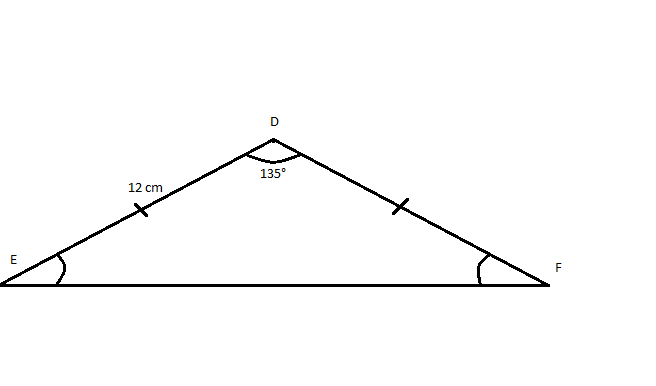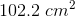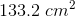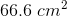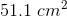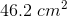Explanation:

The first step to solve for area is to divide the isosceles into two right triangles: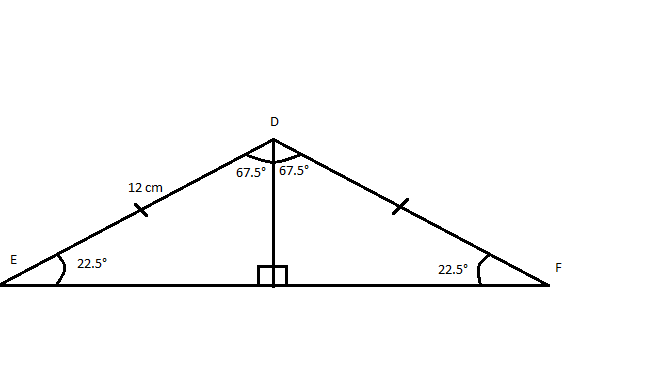From there, we can determine the height and base needed for our area equation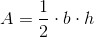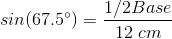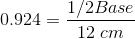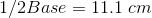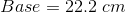From there, height can be easily determined using the Pathegorean Theorem: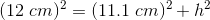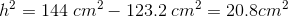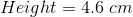Now both values can be plugged into the Area formula: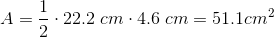Example Question #1 : Solve An Isosceles Triangle By Dividing Into 2 Right Triangles

Find the area of the given isosceles triangle: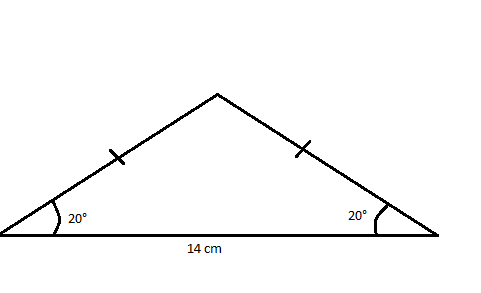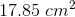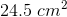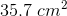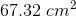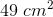Explanation:

The first step is to divide this isosceles triangle into 2 right triangles, making it easier to solve: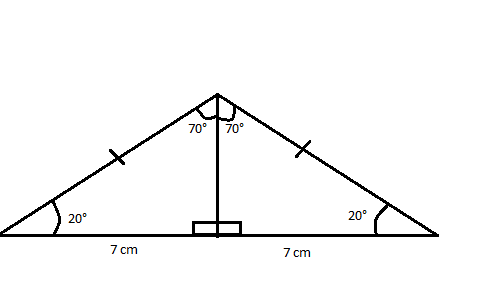The equation for area is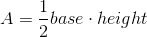We already know the base, so we need to solve for height to get the area.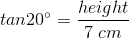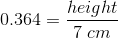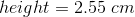Then we plug in all values for the equation: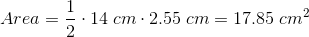Example Question #12 : Trigonometric Applications

Find the area of the given isosceles triangle: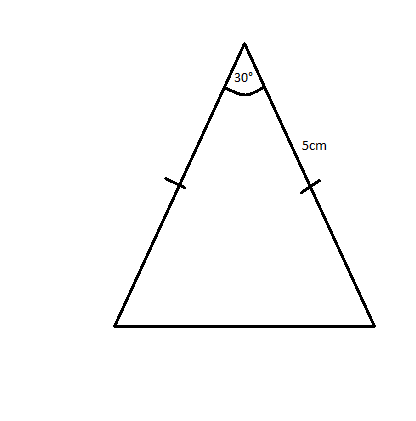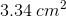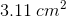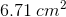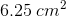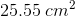Explanation:

The first step toward finding the area is to divide this isosceles triangle into two right triangles: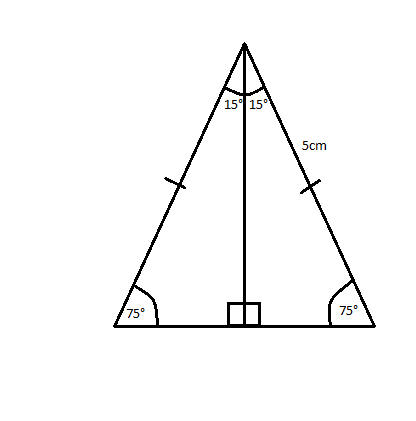Trigonometric ratios can be used to find both the height and the base, which are needed to calculate area: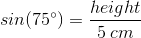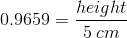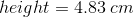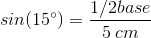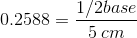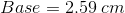With both of those values calculated, we can now calculate the area of the triangle: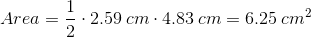All Precalculus Resources